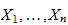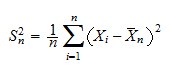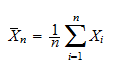StatLect

The unadjusted sample variance measures the average dispersion of a sample of observations around their mean. It is computed by averaging the squared deviations from the mean.## Synonyms

It is also often called biased sample variance, because, under standard assumptions, it is a biased estimator of the population variance.

## Definition

It is defined as follows:

Definition Givenobservations, their unadjusted sample variance is:whereis their sample mean:## Main lecture

The lecture entitled Variance estimation provides a thorough introduction to the concept of unadjusted sample variance, including a detailed analysis of its statistical properties (e.g. biasedness as an estimator of the population variance).

## Related glossary entries

The glossary entry entitled Adjusted sample variance provides a short definition of another - unbiased - estimator of variance.